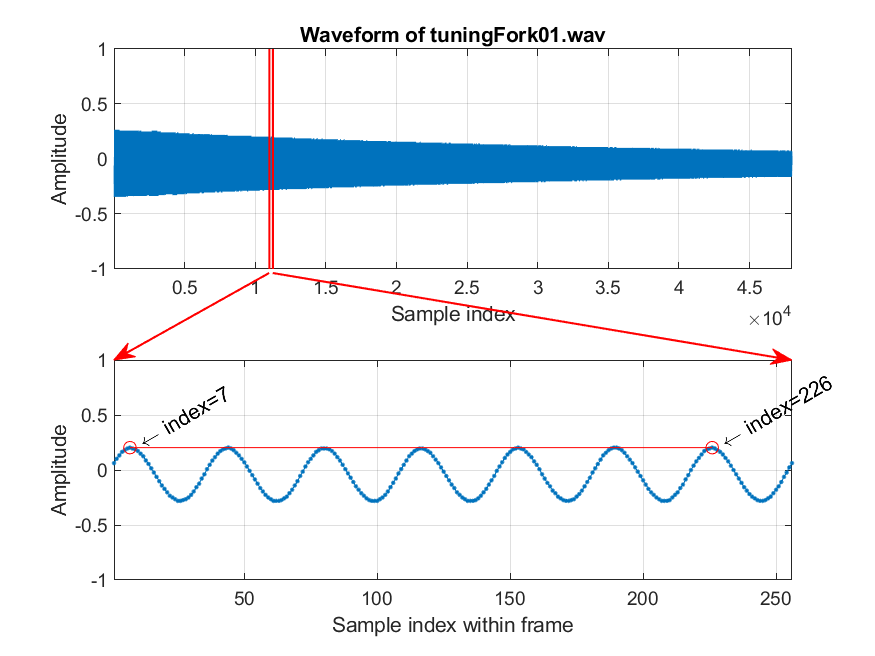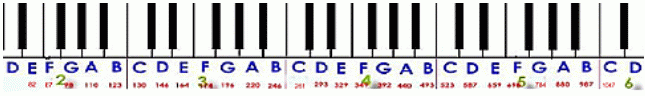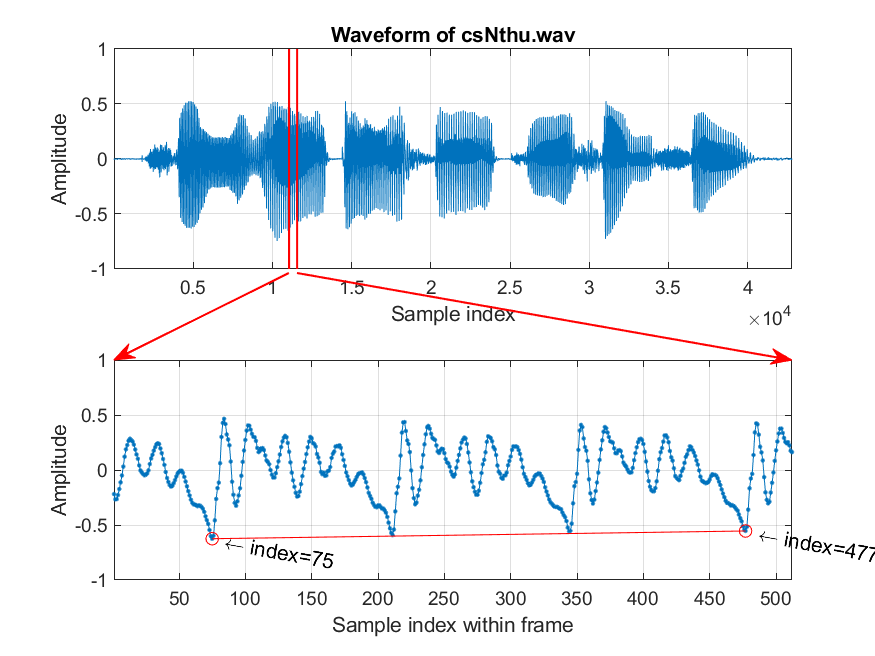## 5-4 Pitch (嚙踝蕭嚙踝蕭嚙踝蕭)

[chinese][all]

Pitch is an important feature of audio signals, especially for quasi-periodic signals such as voiced sounds from human speech/singing and monophonic music from most music instruments. Intuitively speaking, pitch represent the vibration frequency of the sound source of audio signals. In other words, pitch is the fundamental frequency of audio signals, which is equal to the reciprocal of the fundamental period.

Generally speaking, it is not too difficult to observe the fundamental period within a quasi-periodic audio signals. Take a 3-second clip of a tuning fork tuningFork01.wav for example. We can first plot a frame of 256 sample points and identify the fundamental period easily, as shown in the following example.

Example 1: framePitchDisp4tuningFork01.mwaveFile='tuningFork01.wav'; au=myAudioRead(waveFile); y=au.signal; fs=au.fs; index1=11000; frameSize=256; index2=index1+frameSize-1; frame=y(index1:index2); subplot(2,1,1); plot(y); grid on xlabel('Sample index'); ylabel('Amplitude'); title(['Waveform of ', waveFile]); axis([1, length(y), -1 1]); subplot(2,1,2); plot(frame, '.-'); grid on xlabel('Sample index within frame'); ylabel('Amplitude'); point=[7, 226]; % Peaks axis([1, length(frame), -1 1]); periodCount=6; fp=((point(2)-point(1))/periodCount); % fundamental period ff=fs/fp; % fundamental frequency pitch=69+12*log2(ff/440); fprintf('Fundamental period (fp) = (%g-%g)/%g = %g points\n', point(2), point(1), periodCount, fp); fprintf('Fundamental frequency (ff) = %g/%g = %g Hz\n', fs, fp, ff); fprintf('Pitch = %g semitone\n', pitch); % === For plotting arrows, etc % ====== Frame boundary subplot(211); line(index1*[1 1], [-1 1], 'color', 'r', 'linewidth', 1); line(index2*[1 1], [-1 1], 'color', 'r', 'linewidth', 1); % ====== FP coverage subplot(212); line(point, frame(point), 'marker', 'o', 'color', 'red'); % ====== Axis locations subplot(211); loc1=get(gca, 'position'); subplot(212); loc2=get(gca, 'position'); % ====== arrow 1 x1=[loc1(1)+(index1(1)-1)/(length(y)-1)*loc1(3), loc2(1)]; y1=[loc1(2), loc2(2)+loc2(4)]; ah=annotation('arrow', x1, y1, 'color', 'r', 'linewidth', 1); % ======= arrow 2 x2=[loc1(1)+(index2-1)/(length(y)-1)*loc1(3), loc2(1)+loc2(3)]; y2=[loc1(2), loc2(2)+loc2(4)]; ah=annotation('arrow', x2, y2, 'color', 'r', 'linewidth', 1); % ====== Texts indicating start/end indices h1=text(point(1), frame(point(1)), [' \leftarrow index=', int2str(point(1))], 'rotation', 30); h2=text(point(2), frame(point(2)), [' \leftarrow index=', int2str(point(2))], 'rotation', 30); Fundamental period (fp) = (226-7)/6 = 36.5 points Fundamental frequency (ff) = 16000/36.5 = 438.356 Hz Pitch = 68.9352 semitoneIn the above example, the two red lines in the first plot define the start and end of the frame for our analysis. The second plot shows the waveform of the frame as well as two points (identified visually) which cover 5 fundamental periods. Since the distance between these two points is 182 units, the fundamental frequency is fs/(182/5) = 16000/(182/5) = 439.56 Hz, which is equal to 68.9827 semitones. The formula for the conversion from pitch frequency to semitone is shown next.

semitone = 69 + 12*log2(frequency/440)

In other words, when the fundamental frequency is 440 Hz, we have a pitch of 69 semitones, which corresponds to "central la" or A4 in the following piano roll.Hint
The fundamental frequency of the tuning fork is designed to be 440 Hz. Hence the tuning fork are usually used to fine tune the pitch of a piano.

In fact, semitone is also used as unit for specify pitch in MIDI files. From the conversion formula, we can also notice the following facts:

• Each octave contains 12 semitones, including 7 white keys and 5 black ones.
• Each transition to go up one octave corresponds to twice the frequency. For instance, the A4 (central la) is 440 Hz (69 semitones) while A5 is 880 Hz (81 semitones).
• Pitch in terms of semitones (more of less) correlates linearly to human's "perceived pitch".

The waveform of the tuning fork is very "clean" since it is very close to a sinusoidal signal and the fundamental period is very obvious. In the following example, we shall use human's speech as an examle of visual determination of pitch. The clip is my voice of "清華大學資訊系" (csNthu.wav). If we take a frame around the character "華", we can visually identify the fundamental period easily, as shown in the following example.

Example 2: framePitchDisp4speech01.mwaveFile='csNthu.wav'; au=myAudioRead(waveFile); y=au.signal; fs=au.fs; index1=11050; frameSize=512; index2=index1+frameSize-1; frame=y(index1:index2); subplot(2,1,1); plot(y); grid on xlabel('Sample index'); ylabel('Amplitude'); title(['Waveform of ', waveFile]); axis([1, length(y), -1 1]); subplot(2,1,2); plot(frame, '.-'); grid on xlabel('Sample index within frame'); ylabel('Amplitude'); point=[83, 485]; % Peaks point=[75, 477]; % Valleys axis([1, length(frame), -1 1]); periodCount=3; fp=((point(2)-point(1))/periodCount); % fundamental period ff=fs/fp; % fundamental frequency pitch=69+12*log2(ff/440); fprintf('Fundamental period (fp) = (%g-%g)/%g = %g points\n', point(2), point(1), periodCount, fp); fprintf('Fundamental frequency (ff) = %g/%g = %g Hz\n', fs, fp, ff); fprintf('Pitch = %g semitone\n', pitch); % === For plotting arrows, etc % ====== Frame boundary subplot(211); line(index1*[1 1], [-1 1], 'color', 'r', 'linewidth', 1); line(index2*[1 1], [-1 1], 'color', 'r', 'linewidth', 1); % ====== FP coverage subplot(212); line(point, frame(point), 'marker', 'o', 'color', 'red'); % ====== Axis locations subplot(211); loc1=get(gca, 'position'); subplot(212); loc2=get(gca, 'position'); % ====== arrow 1 x1=[loc1(1)+(index1(1)-1)/(length(y)-1)*loc1(3), loc2(1)]; y1=[loc1(2), loc2(2)+loc2(4)]; ah=annotation('arrow', x1, y1, 'color', 'r', 'linewidth', 1); % ======= arrow 2 x2=[loc1(1)+(index2-1)/(length(y)-1)*loc1(3), loc2(1)+loc2(3)]; y2=[loc1(2), loc2(2)+loc2(4)]; ah=annotation('arrow', x2, y2, 'color', 'r', 'linewidth', 1); % ====== Texts indicating start/end indices h1=text(point(1), frame(point(1)), [' \leftarrow index=', int2str(point(1))], 'rotation', -10); h2=text(point(2), frame(point(2)), [' \leftarrow index=', int2str(point(2))], 'rotation', -10); Fundamental period (fp) = (477-75)/3 = 134 points Fundamental frequency (ff) = 16000/134 = 119.403 Hz Pitch = 46.42 semitoneIn the above example, we select a 512-point frame around the vowel of the character "華". In particular, we chose two points (with indices 75 and 477) that covers 3 complete fundamental periods. Since the distance between these two points is 402, the fundamental frequency is fs/(402/3) = 16000/(402/3) = 119.403 Hz and the pitch is 46.420 semitones.

Conceptually, the most obvious sample point within a fundamental period is often referred to as the pitch mark. Usually pitch marks are selected as the local maxima or minima of the audio waveform. In the previous example of pitch determination for the tuning fork, we used two pitch marks that are local maxima. On the other hand, in the example of pitch determination for human speech, we used two pitch marks that are local minima instead since they are more obvious than local maxima. Reliable identification of pitch marks is an essential task for text-to-speech synthesis.

Due to the difference in physiology, the pitch ranges for males ane females are different:

• The pitch range for males is 35 ~ 72 semitones, or 62 ~ 523 Hz.
• The pitch range of females is 45 ~ 83 semitones, or 110 ~ 1000 Hz.

However, it should be emphasized that we are not using pitch alone to identify male or female voices. Moreover, we also use the information from timbre (or more precisely, formants) for such task. More information will be covered in later chapters.

As shown in this section, visual identification of the fundamental frequency is not a difficult task for human. However, if we want to write a program to identify the pitch automatically, there are much more we need to take into consideration. More details will be followed in the next few chapters.

Audio Signal Processing and Recognition (音訊處理與辨識)